#PROPERTIES OF LOGARITHMSProperty 1: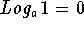because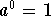.

Example 1: In the equation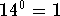, the base is 14 and the exponent is 0. Remember that a logarithm is an exponent, and the corresponding logarithmic equation is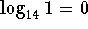where the 0 is the exponent.

Example 2: In the equation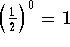, the base is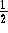and the exponent is 0. Remember that a logarithm is an exponent, and the corresponding logarithmic equation is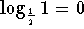.

Example 3: Use the exponential equation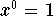to write a logarithmic equation. The base x is greater than 0 and the exponent is 0. The corresponding logarithmic equation is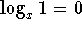.

Property 2: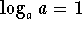because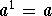.

Example 4: In the equation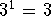, the base is 3, the exponent is 1, and the answer is 3. Remember that a logarithm is an exponent, and the corresponding logarithmic equation is.

Example 5: In the equation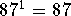, the base is 87, the exponent is 1, and the answer is 87. Remember that a logarithm is an exponent, and the corresponding logarithmic equation is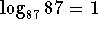.

Example 6: Use the exponential equation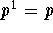to write a logarithmic equation. If the base p is greater than 0, then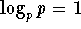.

Property 3: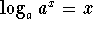because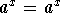.

Example 7: Since you know that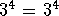, you can write the logarithmic equation with base 3 as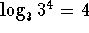.

Example 8: Since you know that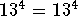, you can write the logarithmic equation with base 13 as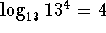.

Example 9: Use the exponential equation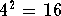to write a logarithmic equation with base 4. You can convert the exponential equationto the logarithmic equation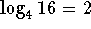. Since the 16 can be written as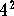, the equationcan be written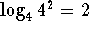.

The above rules are the same for all positive bases. The most common bases are the base 10 and the base e. Logarithms with a base 10 are called common logarithms, and logarithms with a base e are natural logarithms. On your calculator, the base 10 logarithm is noted by log, and the base e logarithm is noted by ln.

There are an infinite number of bases and only a few buttons on your calculator. You can convert a logarithm with a base that is not 10 or e to an equivalent logarithm with base 10 or e. If you are interested in a discussion on how to change the bases of a logarithm, click on Change of Base.

For a discussion of the relationship between the graphs of logarithmic functions and exponential functions, click on graphs.[Algebra] [Trigonometry] [Complex Variables]S.O.S MATHematics home page

Do you need more help? Please post your question on our S.O.S. Mathematics CyberBoard.Author: Nancy Marcus